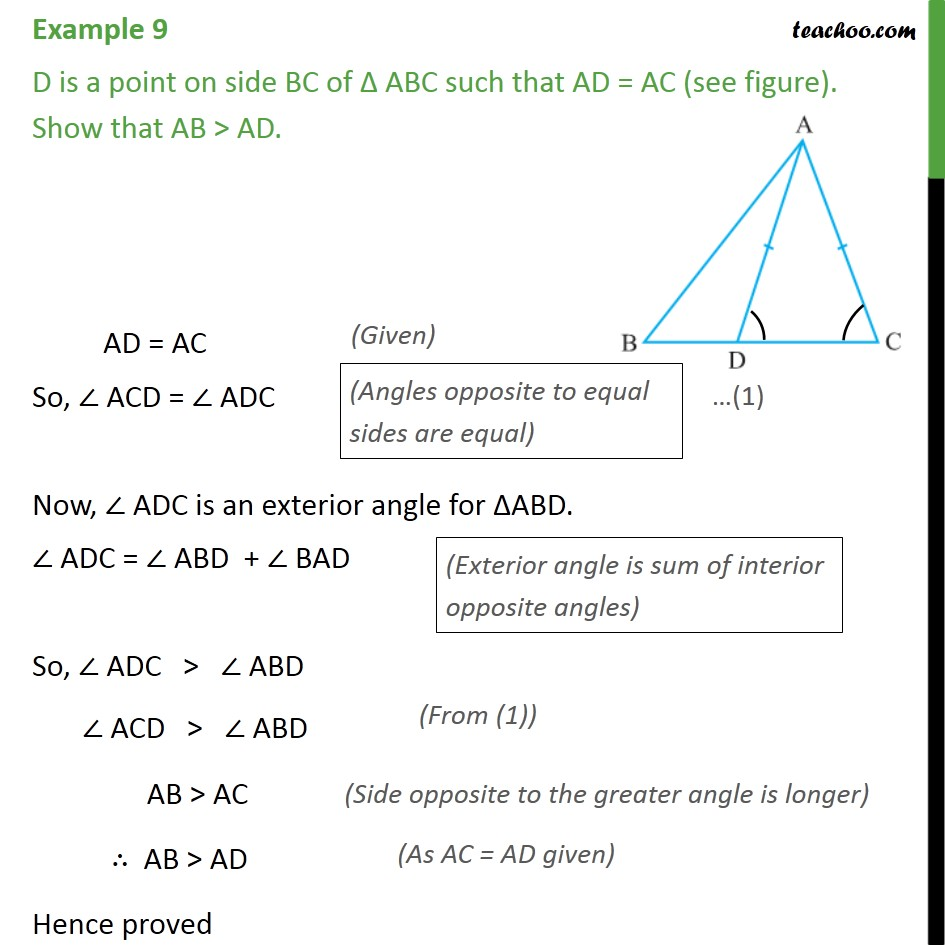1. Chapter 7 Class 9 Triangles (Term 1)
2. Serial order wise
3. Examples

Transcript

Example 9 D is a point on side BC of ABC such that AD = AC (see figure). Show that AB > AD. AD = AC So, ACD = ADC Now, ADC is an exterior angle for ABD. ADC = ABD + BAD So, ADC > ABD ACD > ABD AB > AC AB > AD Hence proved

Examples